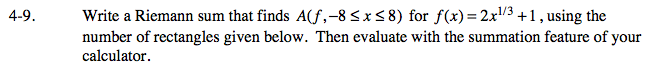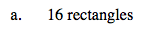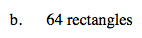### Home > CALC > Chapter 4 > Lesson 4.1.1 > Problem4-9

4-9.
1. Write a Riemann sum that finds A(f, −8 ≤ x ≤ 8) for f(x) = 2x1/3 + 1, using the number of rectangles given below. Then evaluate with the summation feature of your calculator. Homework Help ✎

1. 16 rectangles

2. 64 rectanglesLet's use right endpoint rectangles:Let's use midpoint rectangles:

$\sum_{i=1}^{n-1}\Delta xf\left(a+\frac{\Delta x}{2}i\right)$